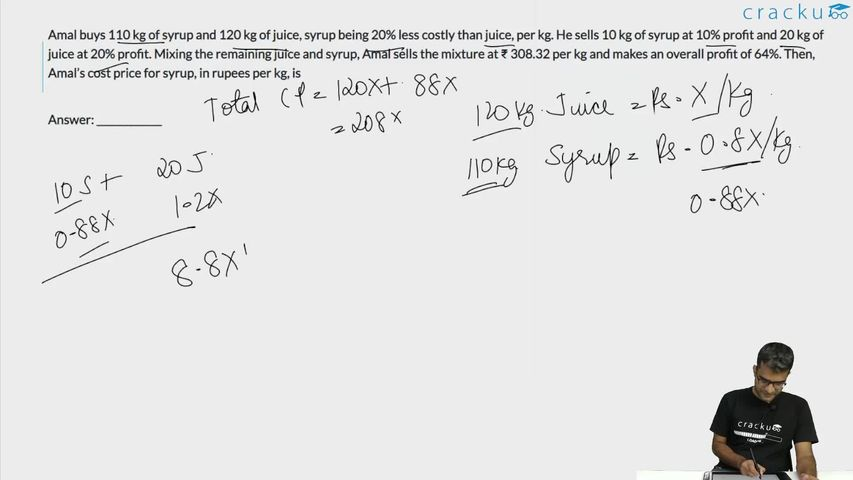Question 12

# Amal buys 110 kg of syrup and 120 kg of juice, syrup being 20% less costly than juice, per kg. He sells 10 kg of syrup at 10% profit and 20 kg of juice at 20% profit. Mixing the remaining juice and syrup, Amal sells the mixture at ₹ 308.32 per kg and makes an overall profit of 64%. Then, Amal’s cost price for syrup, in rupees per kg, is

Solution

Total syrup - 110 kg

Total juice - 120 kg

It is given, cost price of syrup is 20% less than the cost price of juice.

Let the cost price of juice per kg be 10CP

Cost price of syrup per kg is 8CP

10kg syrup -> cost price = 80CP

It is given, 10kg syrup is sold at 10% profit. This implies selling price = 1.1*80CP = 88CP

20kg juice -> cost price = 200CP

It is given, 20kg juice is sold at 20% profit. This implies selling price = 1.2*200CP = 240CP

It is given, Mixing the remaining juice and syrup, Amal sells the mixture at ₹ 308.32 per kg

Selling price of the remaining mixture = 308.32*200 = Rs 61664

Total S.P = 61664 + 328CP

Total C.P = 880CP + 1200CP = 2080CP

Overall profit = 64%

$$61664+328CP=\frac{164}{100}\left(2080CP\right)$$

Solving, we get CP = 20

Cost price for syrup per kg = 8CP = 8*20 = Rs 160

### View Video Solution6 months, 2 weeks ago

0.8 how